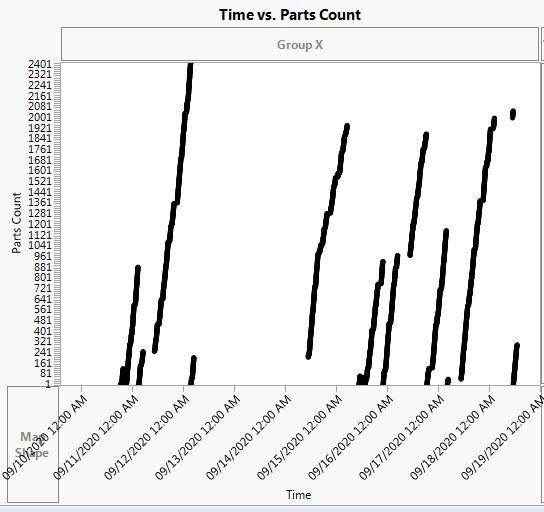Our World Statistics Day conversations have been a great reminder of how much statistics can inform our lives. Do you have an example of how statistics has made a difference in your life? Share your story with the Community!
Choose Language Hide Translation Bar
Highlighted

## Component Count Incrementing

I have data where a certain number of parts are used on a component before it is replaced. At the point when the component is replaced, the part count is reset to 1. I would like to create a column that increments the component count each time the part count is reset to 1. How would I do this?

I attached a picture of the part count over time to help with understanding how the data looks.1 ACCEPTED SOLUTION

Accepted Solutions
Highlighted

## Re: Component Count Incrementing

``````If( Row() == 1,
x = 1,
If( :Part Count == 1,
x++,
x
)
);
x;``````
5 REPLIES 5
Highlighted

## Re: Component Count Incrementing

This will be very easy to do, if you can tell me what is the indicator that the Component has replaced?
Jim
Highlighted

## Re: Component Count Incrementing

The indicator that the component has been replaced is when the part count reverts to 1.

Is this what you needed?

Highlighted

## Re: Component Count Incrementing

Here is a formula that will work

``````If( :Part Count == 1 | Row() == 1,
x = 1,
x
++);
x;``````
Jim
Highlighted

## Re: Component Count Incrementing

It's quite simple. The steps are this:

1. Sort Time column ascending
2. Create new column "ComponentCount" with below formula:
``````New Column("ComponentCount", Numeric, Continuous, Formula(
If(Row() == 1, 0,
:PartCount == 1, Lag(:ComponentCount, 1) + 1
)
)``````

Highlighted

## Re: Component Count Incrementing

``````If( Row() == 1,
x = 1,
If( :Part Count == 1,
x++,
x
)
);
x;``````
Article Labels